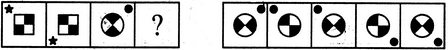# Non Verbal Reasoning - Analogy - Discussion

### Discussion :: Analogy - Section 2 (Q.No.5)

Each of the following questions consists of two sets of figures. Figures A, B, C and D constitute the Problem Set while figures 1, 2, 3, 4 and 5 constitute the Answer Set. There is a definite relationship between figures A and B. Establish a similar relationship between figures C and D by selecting a suitable figure from the Answer Set that would replace the question mark (?) in fig. (D).

5.

Select a suitable figure from the Answer Figures that would replace the question mark (?).(A)     (B)      (C)     (D)                  (1)      (2)      (3)      (4)      (5)

 [A]. 1 [B]. 2 [C]. 3 [D]. 4 [E]. 5

Explanation:

The figure rotates through 90oACW.

 Abhishek said: (Jan 21, 2016) Sir, what is ACW please explain it?

 Savita said: (Jan 30, 2016) Anti clockwise.

 Tarun said: (Feb 11, 2016) If, we go by the logic then the dot is moving anti clockwise and the shaded area within the larger figure has exchanged the position with the unshaded one.

 Vasu said: (Dec 6, 2018) I think 5 is the correct answer. The dot moves up to down in the same side.

 Vicky said: (Aug 2, 2021) I think the Answer is 5.